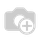# Unpaid Leaves Deduction not workingKeep a new field "month" in hr_payslip model and on saving the record it should be updated as follows,

month = dt.strptime(date_from,'%Y-%m-%d').month

res['value'].update({'month': month})

--------------------------------------
We are using the above month variable to index the days in a month which is given as a list,

result=(contract.wage/[0, 31, 28, 31, 30, 31, 30, 31, 31, 30, 31, 30, 31] [payslip.month])*worked_days.Unpaid and worked_days.Unpaid.number_of_days or Falsehas anyone got the solution , how can we remove 31 with the number of days of the month for which we are calculating the payslip ??This is not answer for question .... when you need to ask a question should put it in new question or comment in the question if you want ..

Sorry for inconvenience, did intentionally to seek attention of the followers of this question.May be i got the answer from them. If u insist ,i may delete this.

# Get the no of days ...........

atej = str(payslip.date_to)

datek = str(payslip.date_from)

dj = datej[-2:]

x=0

new = 0

while (x<=31):

x= x+1

if str(x) == dj or "0"+str(x) == dj:

new= x

dk = datek[-2:]

y=0

old = 0

while (y<=31):

y= y+1

if str(y) == dk or "0"+str(y) == dk:

old= y

no_of_days = new-old + 1

result = no_of_daysFinally I found the solution .. the condition needed for this rule

result=worked_days.Unpaid and worked_days.Unpaid.number_of_days or False

and then working correctly

the following link help in this problem

http://help.openerp.com/question/14645/unpaid-leaves-is-not-decrease-in-the-salary-computation/#14782@Maath Deduction amount is not posibble it is adding...

@sridhar you can make it deduction or adding depending on Salary rule Category and - signal in rule "result=-(contract.wage/31)*worked_days.Unpaid.number_of_days" Now it is working successfully for me any problems with you?

result=worked_days.Unpaid and worked_days.Unpaid.number_of_days or False this concept adding the loss of pay amount.

No, this is a condition for apply the rule will not be Always True , choose python code and put this condition

@Maath Yes Maath now it is working perfectly thank you very much...

This work for me as well. Thanks for the solution @maath . Hats off to you.

@maath : Can we remove 31 with the number of days of the month for which we are calculating the payslip.Do u know the code for that?

Hello Anybody can me tell me how to calculate Overtime in OPenrp